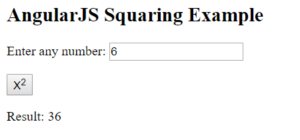# How to Implement Dependency Injection in AngularJsDependency Injection is a software design pattern that specifies the way in which components get hold of their dependencies. The components are given their dependencies instead of hard coding them. Re-usability and maintainability can be achieved by using dependency injection. Supreme Dependency Injection in AngularJs can be done by the following components:

## Value Dependency Injection

A simple object in AngularJs is known as a value. It can be a string, a number or even a JavaScript object. It can be used to pass values in factories, services or controllers during the run and config phase.

Example:

`//define a module  `

`var firstModule = angular.module("firstModule", []);`

`//create a value object and pass data to it  `

`firstModule.value("numberValue", 50);`

`firstModule.value("stringValue", "xyz");`

`firstModule.value("objectValue", { val1 : 456, val2 : "xyz"} );`

In this example, values are defined using the value() function. The name of the value is specified by the first parameter, and the second parameter specifies the value. This enables the factories, services, and controllers to reference these values by their name itself.

Injecting a Value

We can inject a value into the AngularJs controller function by adding a parameter with the same name as the value.

Example:

`var firstModule = angular.module("firstModule", []);`

`firstModule.value("numberValue", 18);`

`firstModule.controller("FirstController", function(\$scope, numberValue) {`

`console.log(numberValue);`

`});`

Learn the latest trends and techniques in UI/UX design through UX Design Course.

## Factory Injection

A function that creates values is known as a factory. A value on demand is created by the factory, whenever a service or controller needs a value injected from the factory. Once the value is created, it is reused for all services and controllers.

It makes usage of the factory function to calculate and return the value.

Example:

`var firstModule = angular.module("firstModule", []);`

`firstModule.factory("firstFactory", function() {`

`return "a value";`

`});`

`firstModule.controller("FirstController", function(\$scope, firstFactory) {`

`console.log(firstFactory);`

`});`

Injecting values into factory

A value can be injected into factory by the following method:

`var firstModule = angular.module("firstModule", []);`

`firstModule.value("numberValue", 29);`

`firstModule.controller("FirstController", function(\$scope, numberValue) {`

`console.log(numberValue);`

`});`

It must be noted that the value produced by the factory function is injected, not the factory function itself. Let’s move on with this article of Dependency Injection in AngularJs.

## Service Injection in AngularJs

A singleton JavaScript object that contains a set of functions is known as a service in AngularJs. The logic necessary for the service to carry out is contained in the function. Service can be created by using the service() function on a module.

Example:

`//define a module  `

`var firstApp = angular.module("firstApp", []);`

`...`

`//create a service which defines a method square to return the square of a number  `

`firstApp.service('CalciService', function(MathService){`

`this.square = function(x) {`

`return MathService.multiply(x,x);`

`}`

`});`

`//inject the service "CalciService" into the controller`

`firstApp.controller('CalciController', function(\$scope, CalciService,`

`defaultInput) {`

`\$scope.number = defaultInput;`

`\$scope.result = CalciService.square(\$scope.number);`

`\$scope.square = function() {`

`\$scope.result = CalciService.square(\$scope.number);`

`}`

`});`

## Provider

To internally create services or factory during the config phase, we make use of Provider. Provider is a special factory method with a get() function which is used to return the value/service/factory.

Example:

`//define a module  `

`var firstApp = angular.module("firstApp", []);`

`...`

`//create a service using provider which defines a method square to return the `

`square of a number.`

`firstApp.config(function(\$provide) {`

`\$provide.provider('MathService', function() {`

`this.\$get = function() {`

`var factory = {};`

`factory.multiply = function(x, y) {`

`return x * y;`

`}`

`return factory;`

`};`

`});`

`});`

## Constant

Since the user cannot inject values into the module.config() function, we make use of constants. Constants are used to pass values at the config phase.

firstApp.constant(“configParam”, “constant value”);

Example:

The directives mentioned above can be used in the following way:

`<html>`

`<head>`

`<title>Dependency Injection</title>`

`</head>`

`<body>`

`<h2>AngularJS Squaring Example</h2>`

`<div ng-app = "firstApp" ng-controller = "CalciController">`

`<p>Enter any number: <input type = "number" ng-model = "number" /></p>`

`<button ng-click = "square()">X<sup>2</sup></button>`

`<p>Result: {{result}}</p>`

`</div>`

`<script src = "https://ajax.googleapis.com/ajax/libs/angularjs/1.3.14/angular.min.js">`

`</script>`

`<script>`

`var firstApp = angular.module("firstApp", []);`

`firstApp.config(function(\$provide) {`

`\$provide.provider('MathService', function() {`

`this.\$get = function() {`

`var factory = {};`

`factory.multiply = function(x, y) {`

`return x * y;`

`}`

`return factory;`

`};`

`});`

`});`

`firstApp.value("defaultInput", 6);`

`firstApp.factory('MathService', function() {`

`var factory = {};`

`factory.multiply = function(x, y) {`

`return x * y;`

`}`

`return factory;`

`});`

`firstApp.service('CalciService', function(MathService) {`

`this.square = function(x) {`

`return MathService.multiply(x,x);`

`}`

`});`

`firstApp.controller('CalciController', function(\$scope, CalciService, defaultInput) {`

`\$scope.number = defaultInput;`

`\$scope.result = CalciService.square(\$scope.number);`

`\$scope.square = function() {`

`\$scope.result = CalciService.square(\$scope.number);`

`}`

`});`

`</script>`

`</body>`

`</html>`

OUTPUT:With this, we come to an end of this Dependency Injection in the AngularJs article. Check out Angular Training Online by Edureka, a trusted online learning company with a network of more than 250,000 satisfied learners spread across the globe.

Got a question for us? Please mention it in the comments section of this Dependency Injection in AngularJs and we will get back to you.

Upcoming Batches For Angular Certification Training Course
Course NameDate
Angular Certification Training Course

Class Starts on 28th October,2023

28th October

SAT&SUN (Weekend Batch)
View DetailsREGISTER FOR FREE WEBINARThank you for registering Join Edureka Meetup community for 100+ Free Webinars each month